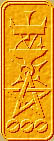Nomography Main > Determinant 4ρ=Σ+Ψ
``` <!-- RegisterNomoPage("determinant04.html") PrintNomoHeader("Reference", "Antique Instruments"); PrintNomoPageNum() //--> ```

# Basic Nomographic Form

The difference between a mere determinant and a nomogram determinant is that the determinant is in Basic Nomographic Form. When it is in BNF the determinant can be used to draw the scales of a nomogram, and the points representing solution values are guaranteed to be on the same straight line. A determinant is in Basic Nomographic Form if all of the following are true:

1. The absolute value of the determinant is zero.
2. Each variable is confined to one row only
3. (though in Grid Nomograms two variables can share a row).
4. The last column must contain only +1.

You don't have to worry about #1, it should have been taken care of by the insertion of the equation into the determinant. #2 is the reason one can use each row to get the X coord and Y coord equations. Obviously if a variable is on two or three rows the ownership of the coord equations is unclear.

So, to confine each variable to a row and to make the last column composed of +1, one uses The Four Rules of Determinants.

## The Four Rules of Determinants

### RULE 1

Rows may be changed into columns and columns into rows.

i.e., one can rotate the determinant around the left diagonal axis. Example:

 A1 B1 C1 A2 B2 C2 A3 B3 C3
=
 A1 A2 A3 B1 B2 B3 C1 C2 C3

### RULE 2

Each element of any row or any column may be multiplied by the same quantity or divided by the same quantity.

Actually, this multiplies the value of the determinant by the quantity. However, since the initial value of the determinant is zero, multiplying it by any quantity will leave it unchanged. Examples:

 A1 B1 C1 A2 B2 C2 A3 B3 C3
=
 A1M B1 C1 A2M B2 C2 A3M B3 C3
 A1 B1 C1 A2 B2 C2 A3 B3 C3
=
 A1 B1 C1 A2 B2 C2 A3/M B3/M C3/M

### RULE 3

Any two rows or any two columns may be interchanged.

Actually this changes the sign of the value of the determinant. However, since the initial value is zero, the sign is unchanged. Examples:

 A1 B1 C1 A2 B2 C2 A3 B3 C3
=
 B1 A1 C1 B2 A2 C2 B3 A3 C3
 A1 B1 C1 A2 B2 C2 A3 B3 C3
=
 A1 B1 C1 A3 B3 C3 A2 B2 C2

### RULE 4

To the elements of any row or column may be added the corresponding elements of any one or more rows or columns. To the elements of any row or column may be added the same multiples of the corresponding elements of any one or more rows or columns.

Examples:

 A1 B1 C1 A2 B2 C2 A3 B3 C3
=
 B1+A1 B1 C1 B2+A2 B2 C2 B3+A3 B3 C3
 A1 B1 C1 A2 B2 C2 A3 B3 C3
=
 A1 B1 C1 A2 B2 C2 3A1+A3 3B1+B3 3C1+C3

Alas, much like factoring, there is no set way to use the Four Rules to convert a determinant into Basic Nomographic Form. One just has to play with it until it works. Of course with experience, one will gain the skill to intuitively see the steps required.

Example 1. Take the following determinant and convert it into Basic Nomographic Form by using the Four Rules.

 1 0 -v 0 1 -w u -1 u2

Using Rule 4, add column 2 to column 1:

 1 0 -v 1 1 -w u-1 -1 u2

Using Rule 2, divide the last row by u-1

 1 0 -v 1 1 -w 1 -1/(u-1) u2/(u-1)

Using Rule 3, interchange column 1 and column 3

 0 -v 1 1 -w 1 -1/(u-1) u2/(u-1) 1

That is in Basic Nomographic Form, but just to be neat one can use Rule 3 and interchange row 1 and row 3:

 -1/(u-1) u2/(u-1) 1 0 -v 1 1 -w 1

Example 2. Take the following determinant and convert it into Basic Nomographic Form by using the Four Rules.

 logV 0 1 logH 1 0 log(π/4)+2logD -1 1

Using Rule 2 multiply right column by 2:

 logV 0 2 logH 1 0 log(π/4)+2logD -1 2

Using Rule 4 add middle column to right column:

 logV 0 2 logH 1 1 log(π/4)+2logD -1 1

Using Rule 2 divide top row by 2:

 logV/2 0 1 logH 1 1 log(π/4)+2logD -1 1

That is in Basic Nomographic Form. However, the parallel scales will be lying on their side instead of upright. Turn them upright by using Rule 3 and interchanging the first and middle columns

 0 logV/2 1 1 logH 1 -1 log(π/4)+2logD 1

Example 3. Take the following determinant and convert it into Basic Nomographic Form by using the Four Rules. (Note: " √ " should be a square root symbol)

 √E V/8.03 0 0 1 1 -√W 0 1

Using Rule 2 divide the middle column by V/8.03. This separates the variables into individual rows:

 √E 1 0 0 8.03/V 1 -√W 0 1

Using Rule 4 add the middle column to the right column to adjust the upper right element:

 √E 1 1 0 8.03/V 1+(8.03/V) -√W 0 1

Using Rule 2 divide the middle row by 1+(8.03/v):

 √E 1 1 0 (8.03/V) / (1+(8.03/V)) 1 -√W 0 1

That is in Basic Nomographic Form.